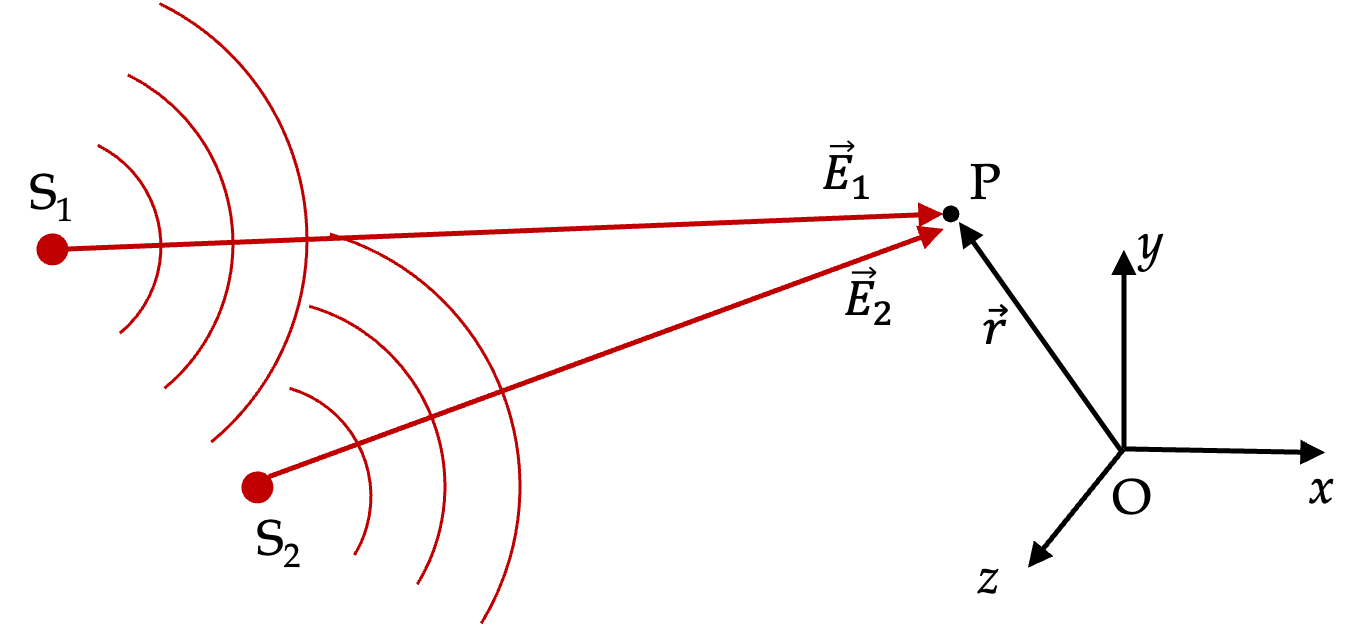## Section50.1Superposition Principle and Interference

In your studies you have seen quite a few superposition principles. For instance, superposition of forces tells us that net force is the vector sum of all the forces.

\begin{equation*} \vec F_\text{net} = \vec F_1 + \vec F_2 + \cdots + \vec F_N. \end{equation*}

Similarly, you saw that vector sum of electric fields at a point gives the actual electric field at that point.

\begin{equation*} \vec E = \vec E_1 + \vec E_2 + \cdots + \vec E_N. \end{equation*}

Similarly for superposition of magnetic fields.

\begin{equation*} \vec B = \vec B_1 + \vec B_2 + \cdots + \vec B_N. \end{equation*}

Since light consists of oscillating electric and magnetic fields, it will not come as a surprise that if you have multiple waves, then the net wave will be sum of the individual waves.

For illustrative purposes, suppose we have two sources of light at $\text{S}_1$ and $\text{S}_2$ as shown Figure 50.1.1 and we want to know the wave at some point P whose position is $\vec r\text{.}$ Let the electric field waves from $\text{S}_1$ and $\text{S}_2$ at P be denoted by $\vec E_1(\vec r, t)$ and $\vec E_2(\vec r, t)\text{,}$ respectively. Then, the net electric field of the resulting wave at P will be

\begin{equation} \vec E(\vec r, t) = \vec E_1(\vec r, t) + \vec E_2(\vec r, t).\tag{50.1.1} \end{equation}Figure 50.1.1. Superposition of two waves illustrated. The waves from sources $\text{S}_1$ and $\text{S}_2$ overlap in space. What will be the wave at point P?

So, why is this a big deal? The big deal comes from the fact that we do not observe these fields, but only the intensity of the resulting wave. Now, you may recall that intensity is related to the time average of the square of the wave. In vector notation, intensity turns out to be

\begin{equation} I = c\epsilon_0 \langle \vec E \cdot \vec E \rangle,\label{eq-intensity-in-terms-of-electric-field-wave}\tag{50.1.2} \end{equation}

where $\langle ... \rangle$ denotes time average of the quantity enclosed. Let $I_1$ and $I_2$ denote the intensities from souorces $\text{S}_1$ and $\text{S}_2\text{.}$ That is,

\begin{equation} I_1 = c\epsilon_0 \langle \vec E_1 \cdot \vec E_1 \rangle,\ \ I_2 = c\epsilon_0 \langle \vec E_2 \cdot \vec E_2 \rangle.\tag{50.1.3} \end{equation}

Now, using $\vec E = \vec E_1 + \vec E_2$ in Eq. (50.1.2) we see that net intensity at P will not equal just the sum of the intensities but it will also have other contributions.

\begin{equation} I = I_1 + I_2 + 2 c\epsilon_0 \langle \vec E_1 \cdot \vec E_2 \rangle.\tag{50.1.4} \end{equation}

The third term on the right side is called the interference term. Since the dot product of two vectors can be zero, positive, or negative, this term will either have no effect at P, or give more intensity that just the sum of the intensities, or have lower intensity than the sum, respectively.

This variation of intensity in space is said to be due to interference between the two waves and the resulting pattern of brightness and darkness is called interference pattern. When intensity at P is less than $I_1 + I_2\text{,}$ we say that there is a desctructive interference there and when intensity at P is more than $I_1 + I_2\text{,}$ we say that there is constructive interference there.

Interference pattern is very sensitive to the distances from the sources. The change in interference can be used to detect and measure small distances, which can be of the order of the wavelength of light. For instance, when using visible light, it is possible to get sub-micron distances by interference quite easily.